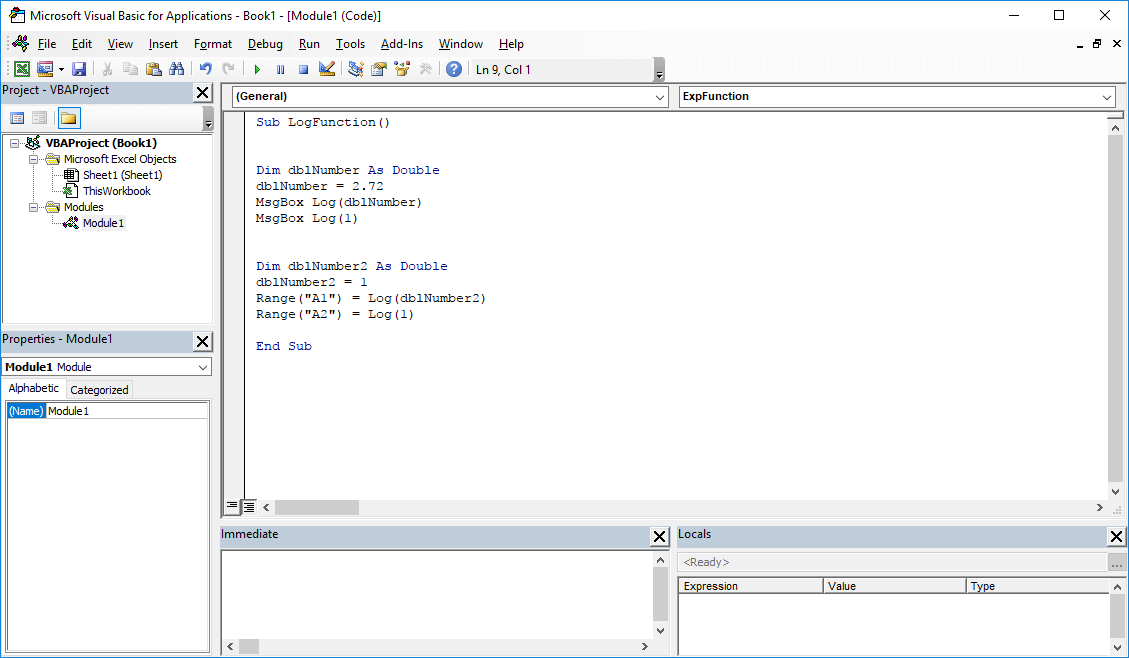# Log VBA function. How to calculate the natural logarithm

## 1. Log VBA function – Description

The Log VBA function returns value of the natural logarithm. Natural logarithm is based on e number ~ 2.72 (Euler’s number). To calculate natural logarithm value, you should use this function. For example, the value of natural logarithm from 1 is 0.

## 2. VBA Log function – Syntax

```'officeinside.org
Log (Number As Double) As Double```

Number: A numeric value used to calculate value of the natural logarithm.

Function return: Double / numeric value.

## 3. VBA Log function – Example

How to use Log  function in VBA Excel? Here are examples of using the Log function in the VisualBasic Editor.

Example 1: Example of using the Log VBA function – result in the MsgBox window.

```'officeinside.org
Dim dblNumber As Double
dblNumber = 1
MsgBox Log (dblNumber)
MsgBox Log (1)```

Example 2: VBA Log – an example of a function – result in Excel spreadsheet.

```'officeinside.org
Dim dblNumber2 As Double
dblNumber2 = 1
Range ("A1") = Log (dblNumber2)
Range ("A2") = Log (1)```Excel VBA functions – Log VBA function

• none

## 5. Log VisualBasic function – Where to use?

The function can be used in: Excel 2003, Excel 2007, Excel 2010, Excel 2013, Excel 2016.

This article is part of the list of VBA Excel functions. A list of all VBA functions can be found at this address: Excel VBA functions.